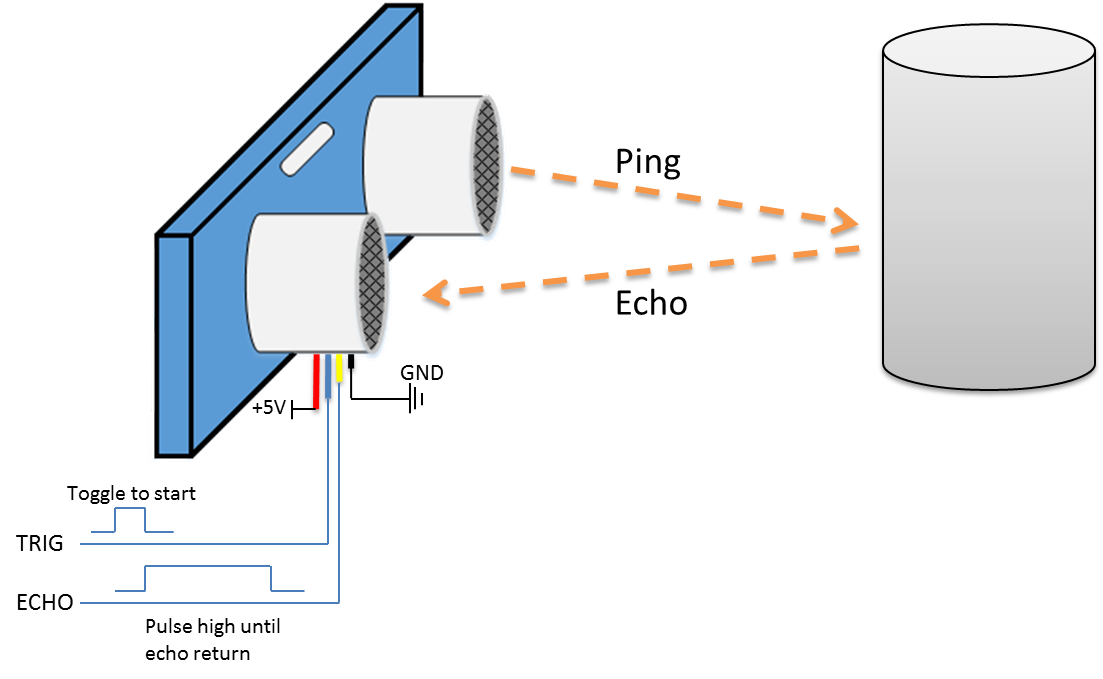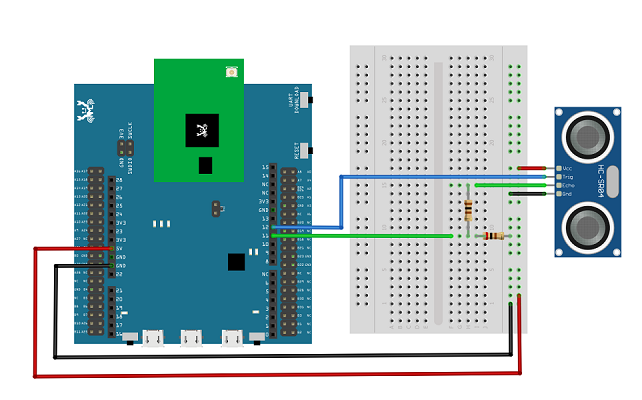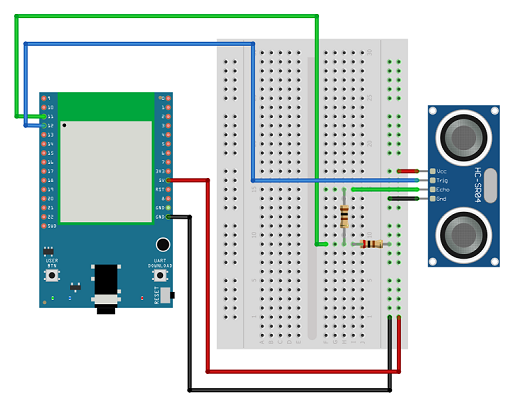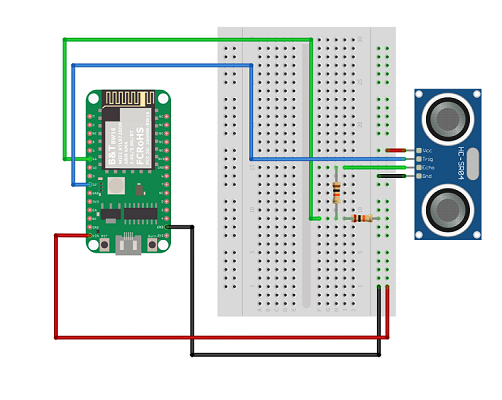# Ameba Arduino: [RTL8722CSM] [RTL8722DM] GPIO - Measure the Distance by Ultrasound Module

Status
Not open for further replies.

#### iot_crab

##### NewbiePreparation
• Ameba x 1
• HC-SR04 Ultrasonic x 1
• Dropping resistor or Level converter
Example
HC-SR04 is a module that uses ultrasound to measure the distance. It looks like a pair of eyes in its appearance, therefore it’s often installed onto robot-vehicle or mechanical bugs to be their eyes. The way it works is that first we “toggle high” the TRIG pin (that is to pull high then pull low). The HC-SR04 would send eight 40kHz sound wave signal and pull high the ECHO pin. When the sound wave returns back, it pull low the ECHO pin.Assume the velocity of sound is 340 m/s, the time it takes for the sound to advance 1 cm in the air is 340*100*10^-6 = 29 us。 The sound wave actually travels twice the distance between HC-SR04 and the object, therefore the distance can be calculated by (time/29) / 2 = time / 58。 The working voltage of HC-SR04 is 5V. When we pull high the ECHO pin to 5V, the voltage might cause damage to the GPIO pin of Ameba. To avoid this situation, we need to drop the voltage as follows:We pick the resistors with resistance 1:2, in the example we use 10kΩ and 20kΩ. If you do not have resistors in hand, you can use level converter instead.The TXB0108 8 channel level converter
is a suitable example:

Next, open the sample code in “File” -> “Examples” -> “AmebaGPIO” -> “HCSR04_Ultrasonic”Compile and upload to Ameba, then press the reset button. Open the Serial Monitor, the calculated result is output to serial monitor every 2 seconds.Note that the HCSR04 module uses the reflection of sound wave to calculate the distance, thus the result can be affected by the surface material of the object (e.g., harsh surface tends to cause scattering of sound wave, and soft surface may cause the sound wave to be absorbed).
Code Reference
Before the measurement starts, we need to pull high the TRIG pin for 10us and then pull low. By doing this, we are telling the HC-SR04 that we are about to start the measurement:

Code:
digitalWrite(trigger_pin, HIGH);
delayMicroseconds(10);
digitalWrite(trigger_pin, LOW);

Next, use pulseIn to measure the time when the ECHO pin is pulled high.duration = pulseIn (echo_pin, HIGH);

Finally, use the formula to calculate the distance.distance = duration / 58;

If you would like to know more about the BW16 development board, please refer to the websites below:
1. Introduction of RTL8720DN on Instructable:
RTL8720DN
2. Load Arduino image into BW16:
How to load BW16 program with Arduino - #13 by Alphi_Jiang
4. B&T “RTL8720D AT Command User Manual”:
https://docs.ai-thinker.com/_media/rtl8710/docs/rtl8720d-at指令手册v2.4.1-20190814.pdf